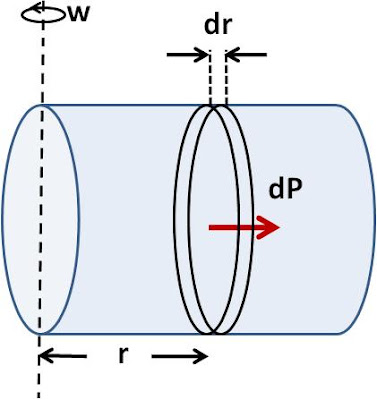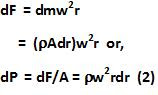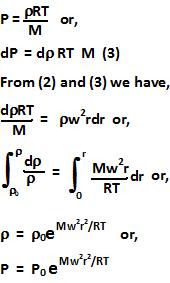## Sunday, February 21, 2010

### Irodov Problem 2.20Consider an infinitesimally thin section of the cylinder of width dr at a distance r from the axis of rotation. Let the area of cross-section of the cylinder be A. Let the density of the gas within this infinitesimally thin section of the cylinder be. The the total mass on gas contained in the infinitesimally thin section of the cylinder will be,The centrifugal force exerted by this infinitesimally thin section of the gas on the rest of the gas (for the gas with distance greater than r from the axis) will be,From the ideal gas law equation we know that,